Learn data science and machine learning by building real-world projects on Jovian

## Data Visualization using Python, Matplotlib and Seaborn

#### Part 8 of "Data Analysis with Python: Zero to Pandas"

This tutorial is the eighth in a series on introduction to programming and data analysis using the Python language. These tutorials take a practical coding-based approach, and the best way to learn the material is to execute the code and experiment with the examples. Check out the full series here:

### How to run the code

This tutorial hosted on Jovian.ml, a platform for sharing data science projects online. You can "run" this tutorial and experiment with the code examples in a couple of ways: using free online resources (recommended) or on your own computer.

This tutorial is a Jupyter notebook - a document made of "cells", which can contain explanations in text or code written in Python. Code cells can be executed and their outputs e.g. numbers, messages, graphs, tables, files etc. can be viewed within the notebook, which makes it a really powerful platform for experimentation and analysis. Don't afraid to experiment with the code & break things - you'll learn a lot by encoutering and fixing errors. You can use the "Kernel > Restart & Clear Output" menu option to clear all outputs and start again from the top of the notebook.

#### Option 1: Running using free online resources (1-click, recommended)

The easiest way to start executing this notebook is to click the "Run" button at the top of this page, and select "Run on Binder". This will run the notebook on mybinder.org, a free online service for running Jupyter notebooks. You can also select "Run on Colab" or "Run on Kaggle", but you'll need to create an account on Google Colab or Kaggle to use these platforms.

#### Option 2: Running on your computer locally

You'll need to install Python and download this notebook on your computer to run in locally. We recommend using the Conda distribution of Python. Here's what you need to do to get started:

1. Install Conda by following these instructions. Make sure to add Conda binaries to your system `PATH` to be able to run the `conda` command line tool from your Mac/Linux terminal or Windows command prompt.

2. Create and activate a Conda virtual environment called `zerotopandas` which you can use for this tutorial series:

``````conda create -n zerotopandas -y python=3.8
conda activate zerotopandas
``````

You'll need to create the environment only once, but you'll have to activate it every time want to run the notebook. When the environment is activated, you should be able to see a prefix `(python-matplotlib-data-visualization)` within your terminal or command prompt.

1. Install the required Python libraries within the environmebt by the running the following command on your terminal or command prompt:
``````pip install jovian jupyter numpy pandas matplotlib seaborn --upgrade
``````
1. Download the notebook for this tutorial using the `jovian clone` command:
``````jovian clone aakashns/python-matplotlib-data-visualization
``````

The notebook is downloaded to the directory `python-matplotlib-data-visualization`.

1. Enter the project directory and start the Jupyter notebook:
``````cd python-matplotlib-data-visualization
jupyter notebook
``````
1. You can now access Jupyter's web interface by clicking the link that shows up on the terminal or by visiting http://localhost:8888 on your browser. Click on the notebook `python-matplotlib-data-visualization.ipynb` to open it and run the code. If you want to type out the code yourself, you can also create a new notebook using the "New" button.

### Introduction

Data visualization is the graphic representation of data. It involves producing images that communicate relationships among the represented data to viewers. Visualizing data is an essential part of data analysis and machine learning. In this tutorial, we'll use Python libraries Matplotlib and Seaborn to learn and apply some popular data visualization techniques.

To begin let's import the libraries. We'll use the `matplotlib.pyplot` for basic plots like line & bar charts. It is often imported with the alias `plt`. The `seaborn` module will be used for more advanced plots, and it is imported with the alias `sns`.

In :
``````# Uncomment the next line to install the required libraries
# !pip install matplotlib seaborn --upgrade --quiet``````
In :
``````import matplotlib.pyplot as plt
import seaborn as sns
%matplotlib inline
``````

Notice this we also include the special command `%matplotlib inline` to ensure that plots are shown and embedded within the Jupyter notebook itself. Without this command, sometimes plots may show up in pop-up windows.

### Line Chart

Line charts are one of the simplest and most widely used data visualization techniques. A line chart displays information as a series of data points or markers, connected by straight lines. You can customize the shape, size, color and other aesthetic elements of the markers and lines for better visual clarity.

Here's a Python list showing the yield of apples (tons per hectare) over 6 years in an imaginary country called Kanto.

In :
``yield_apples = [0.895, 0.91, 0.919, 0.926, 0.929, 0.931]``

We can visualize how the yield of apples changes over time using a line chart. To draw a line chart, we can use the `plt.plot` function.

In :
``plt.plot(yield_apples)``
Out:
``[<matplotlib.lines.Line2D at 0x7f92e88a5640>]``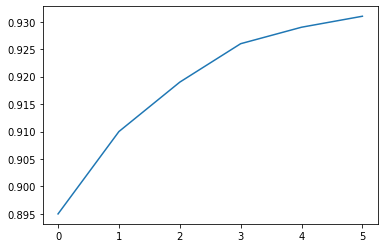Calling the `plt.plot` function draws the line chart as expected, and also returns a list of plots drawn `[<matplotlib.lines.Line2D at 0x7ff70aa20760>]` shown within the output. We can include a semicolon (`;`) at the end of the last statement in the cell to avoiding showing the output and just display the graph.

In :
``plt.plot(yield_apples);``Let's enhance this plot step-by-step to make it more informative and beautiful.

#### Customizing the X-axis

The X-axis of the plot currently shows list element indexes 0 to 5. The plot would be more informative if we could show the year for which the data is being plotted. We can do this by two arguments `plt.plot`.

In :
``````years = [2010, 2011, 2012, 2013, 2014, 2015]
yield_apples = [0.895, 0.91, 0.919, 0.926, 0.929, 0.931]``````
In :
``plt.plot(years, yield_apples)``
Out:
``[<matplotlib.lines.Line2D at 0x7f92e8a6e0d0>]``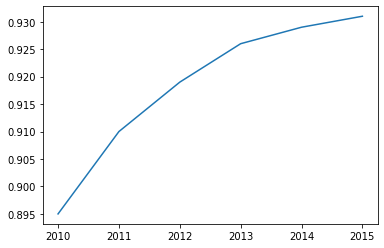#### Axis Labels

We can add labels to the axes to show what each axis represents using the `plt.xlabel` and `plt.ylabel` methods.

In :
``````plt.plot(years, yield_apples)
plt.xlabel('Year')
plt.ylabel('Yield (tons per hectare)');``````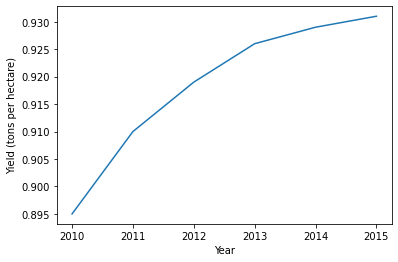#### Plotting Multiple Lines

It's really easy to plot multiple lines in the same graph. Just invoke the `plt.plot` function multiple times. Let's compare the yields of apples vs. oranges in Kanto.

In :
``````years = range(2000, 2012)
apples = [0.895, 0.91, 0.919, 0.926, 0.929, 0.931, 0.934, 0.936, 0.937, 0.9375, 0.9372, 0.939]
oranges = [0.962, 0.941, 0.930, 0.923, 0.918, 0.908, 0.907, 0.904, 0.901, 0.898, 0.9, 0.896, ]``````
In :
``````plt.plot(years, apples)
plt.plot(years, oranges)
plt.xlabel('Year')
plt.ylabel('Yield (tons per hectare)');``````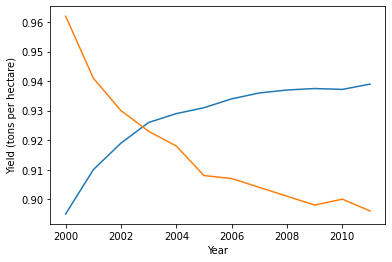#### Chart Title and Legend

To differentiate between multiple lines, we can include a legend within the graph using the `plt.legend` function. We also give the entire chart a title using the `plt.title` function.

In :
``````plt.plot(years, apples)
plt.plot(years, oranges)

plt.xlabel('Year')
plt.ylabel('Yield (tons per hectare)')

plt.title("Crop Yields in Kanto")
plt.legend(['Apples', 'Oranges']);``````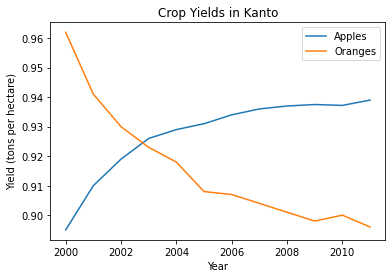#### Line Markers

We can also show markers for the data points on each line using the `marker` argument of `plt.plot`. Matplotlib supports many different types of markers like circle, cross, square, diamond etc. You can find the full list of marker types here: https://matplotlib.org/3.1.1/api/markers_api.html

In :
``````plt.plot(years, apples, marker='o')
plt.plot(years, oranges, marker='x')

plt.xlabel('Year')
plt.ylabel('Yield (tons per hectare)')

plt.title("Crop Yields in Kanto")
plt.legend(['Apples', 'Oranges']);``````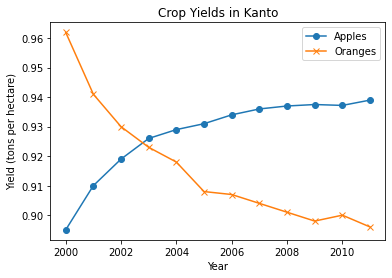#### Styling lines and markers

The `plt.plot` function supports many arguments for styling lines and markers:

• `color` or `c`: set the color of the line (supported colors)
• `linestyle` or `ls`: choose between a solid or dashed line
• `linewidth` or `lw`: set the width of a line
• `markersize` or `ms`: set the size of markers
• `markeredgecolor` or `mec`: set the edge color for markers
• `markeredgewidth` or `mew`: set the edge width for markers
• `markerfacecolor` or `mfc`: set the fill color for markers
• `alpha`: opacity of the plot

Check out the documentation for `plt.plot` to learn more: https://matplotlib.org/api/_as_gen/matplotlib.pyplot.plot.html#matplotlib.pyplot.plot

In :
``````plt.plot(years, apples, marker='s', c='b', ls='-', lw=2, ms=8, mew=2, mec='navy')
plt.plot(years, oranges, marker='o', c='r', ls='--', lw=3, ms=10, alpha=.5)

plt.xlabel('Year')
plt.ylabel('Yield (tons per hectare)')

plt.title("Crop Yields in Kanto")
plt.legend(['Apples', 'Oranges']);``````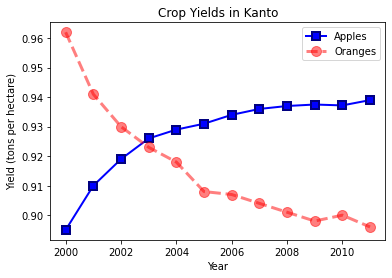The `fmt` argument provides a shorthand for specifying the line style, marker and line color. It can be provided as the third argument to `plt.plot`.

``````fmt = '[marker][line][color]'
``````
In :
``````plt.plot(years, apples, 's-b')
plt.plot(years, oranges, 'o--r')

plt.xlabel('Year')
plt.ylabel('Yield (tons per hectare)')

plt.title("Crop Yields in Kanto")
plt.legend(['Apples', 'Oranges']);``````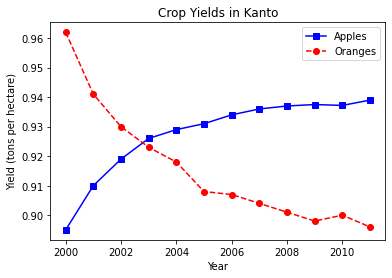If no line style is specified in `fmt`, only markers are drawn.

In :
``````plt.plot(years, oranges, 'or')
plt.title("Yield of Oranges (tons per hectare)");``````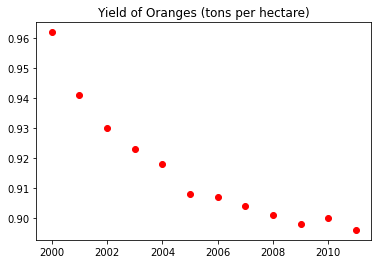#### Changing the Figure Size

You can use the `plt.figure` function to change the size of the figure.

In :
``````plt.figure(figsize=(12, 6))

plt.plot(years, oranges, 'or')
plt.title("Yield of Oranges (tons per hectare)");``````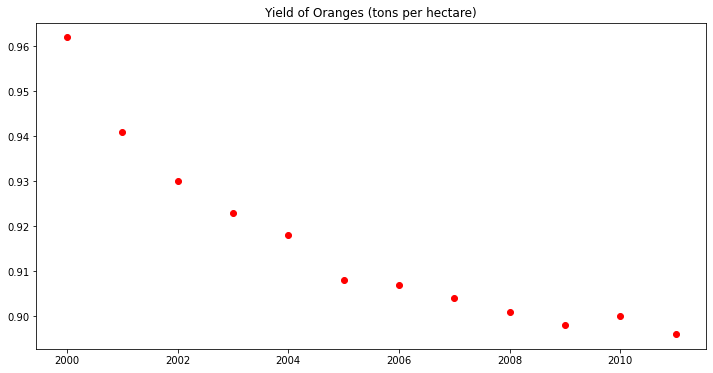#### Improving Default Styles using Seaborn

An easy way to make your charts look beautiful is to use some default styles provided in the Seaborn library. These can be applied globally using the `sns.set_style` function. You can see a full list of predefined styles here: https://seaborn.pydata.org/generated/seaborn.set_style.html

In :
``sns.set_style("whitegrid")``
In :
``````plt.plot(years, apples, 's-b')
plt.plot(years, oranges, 'o--r')

plt.xlabel('Year')
plt.ylabel('Yield (tons per hectare)')

plt.title("Crop Yields in Kanto")
plt.legend(['Apples', 'Oranges']);``````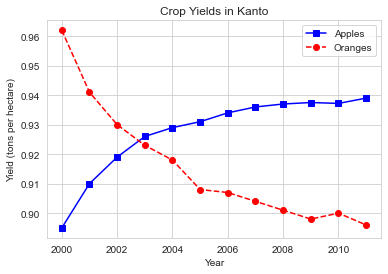In :
``sns.set_style("darkgrid")``
In :
``````plt.plot(years, apples, 's-b')
plt.plot(years, oranges, 'o--r')

plt.xlabel('Year')
plt.ylabel('Yield (tons per hectare)')

plt.title("Crop Yields in Kanto")
plt.legend(['Apples', 'Oranges']);``````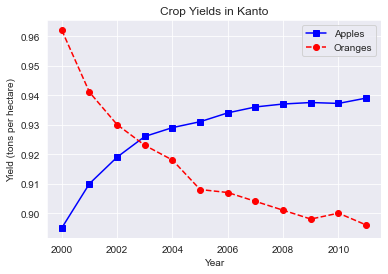In :
``````plt.plot(years, oranges, 'or')
plt.title("Yield of Oranges (tons per hectare)");``````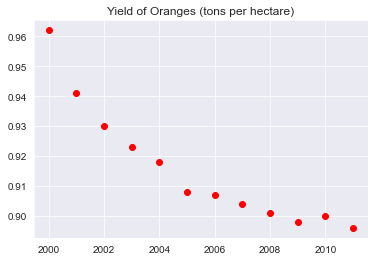You can also edit default styles directly by modifying the `matplotlib.rcParams` dictionary. Learn more: https://matplotlib.org/3.2.1/tutorials/introductory/customizing.html#matplotlib-rcparams

In :
``import matplotlib``
In :
``````matplotlib.rcParams['font.size'] = 14
matplotlib.rcParams['figure.figsize'] = (9, 5)
matplotlib.rcParams['figure.facecolor'] = '#00000000'``````

Whether you're running this Jupyter notebook on an online service like Binder or on your local machine, it's important to save your work from time, so that you can access it later, or share it online. You can upload this notebook to your Jovian.ml account using the `jovian` Python library.

In :
``````# Install the library
``````
In :
``import jovian``
In :
``jovian.commit(project='python-matplotlib-data-visualization')``
```[jovian] Attempting to save notebook.. [jovian] Updating notebook "aakashns/python-matplotlib-data-visualization" on https://jovian.ml/ [jovian] Uploading notebook.. [jovian] Capturing environment.. [jovian] Committed successfully! https://jovian.ml/aakashns/python-matplotlib-data-visualization ```
Out:
``'https://jovian.ml/aakashns/python-matplotlib-data-visualization'``

### Scatter Plot

In a scatter plot, the values of 2 variables are plotted as points on a 2-dimensional grid. Additionally, you can also use a third variable to determine the size or color of the points. Let's try out an example.

The Iris flower dataset provides samples measurements of sepals and petals for 3 species of flowers. The Iris dataset is included with the Seaborn library, and can be loaded as a Pandas data frame.

In :
``````# Load data into a Pandas dataframe
In :
``flowers_df``
Out:
In :
``flowers_df.species.unique()``
Out:
``array(['setosa', 'versicolor', 'virginica'], dtype=object)``

Let's try to visualize the relationship between sepal length and sepal width. Our first instinct might be to create a line chart using `plt.plot`. However, the output is not very informative as there are too many combinations of the two properties within the dataset, and there doesn't seem to be simple relationship between them.

In :
``plt.plot(flowers_df.sepal_length, flowers_df.sepal_width);``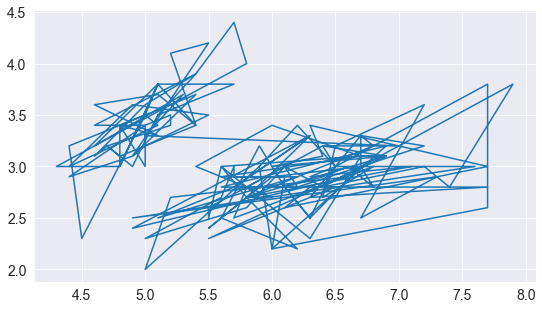We can use a scatter plot to visualize how sepal length & sepal width vary using the `scatterplot` function from `seaborn` (imported as `sns`).

In :
``sns.scatterplot(flowers_df.sepal_length, flowers_df.sepal_width);``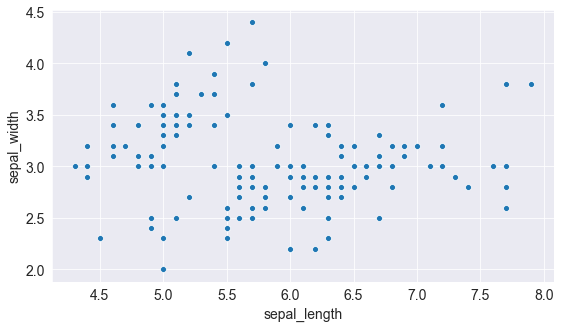Notice how the points in the above plot seem to form distinct clusters with some outliers. We can color the dots using the flower species as a `hue`. We can also make the points larger using the `s` argument.

In :
``sns.scatterplot(flowers_df.sepal_length, flowers_df.sepal_width, hue=flowers_df.species, s=100);``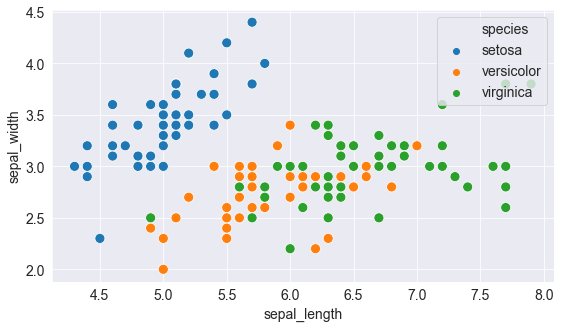Adding hues makes the plot more informative. We can immediately tell that flowers of the Setosa species have a smaller sepal length but higher sepal widths, while the opposite holds true for the Virginica species.

#### Customizing Seaborn Figures

Since Seaborn uses Matplotlib's plotting functions internally, we can use functions like `plt.figure` and `plt.title` to modify the figure.

In :
``````plt.figure(figsize=(12, 6))
plt.title('Sepal Dimensions')

sns.scatterplot(flowers_df.sepal_length,
flowers_df.sepal_width,
hue=flowers_df.species,
s=100);``````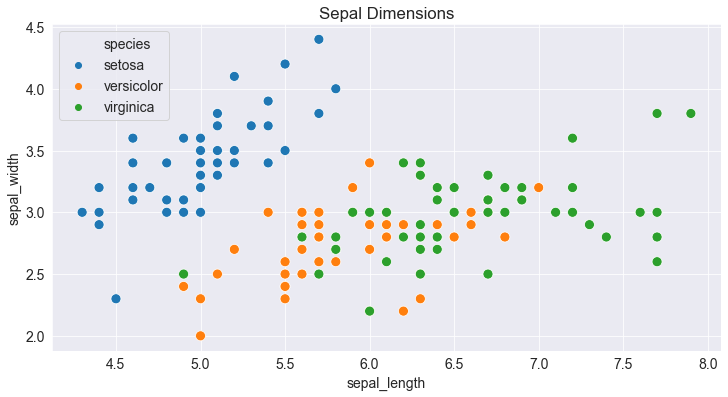#### Plotting using Pandas Data Frames

Seaborn has in-built support for Pandas data frames. Instead of passing each column as a series, you can also pass column names and use the `data` argument to pass the data frame.

In :
``````plt.title('Sepal Dimensions')
sns.scatterplot('sepal_length',
'sepal_width',
hue='species',
s=100,
data=flowers_df);
``````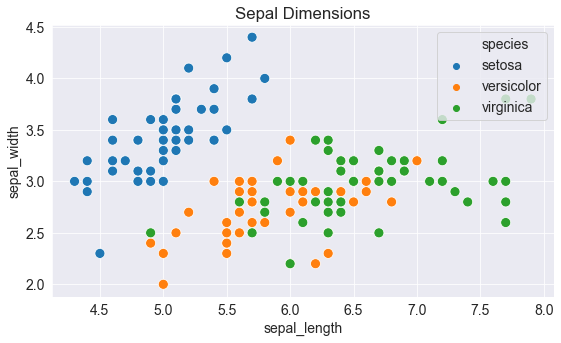Let's save and upload our work before continuing.

In :
``import jovian``
In :
``jovian.commit()``
```[jovian] Attempting to save notebook.. [jovian] Updating notebook "aakashns/python-matplotlib-data-visualization" on https://jovian.ml/ [jovian] Uploading notebook.. [jovian] Capturing environment.. [jovian] Committed successfully! https://jovian.ml/aakashns/python-matplotlib-data-visualization ```
Out:
``'https://jovian.ml/aakashns/python-matplotlib-data-visualization'``

### Histogram

A histogram represents the distribution of data by forming bins along the range of the data and then drawing bars to show the number of observations that fall in each bin.

As an example, let's visualize the how the values of sepal width in the flowers dataset are distributed. We can use the `plt.hist` function to create a histogram.

In :
``````# Load data into a Pandas dataframe
In :
``flowers_df.sepal_width``
Out:
``````0      3.5
1      3.0
2      3.2
3      3.1
4      3.6
...
145    3.0
146    2.5
147    3.0
148    3.4
149    3.0
Name: sepal_width, Length: 150, dtype: float64``````
In :
``````plt.title("Distribution of Sepal Width")
plt.hist(flowers_df.sepal_width);
``````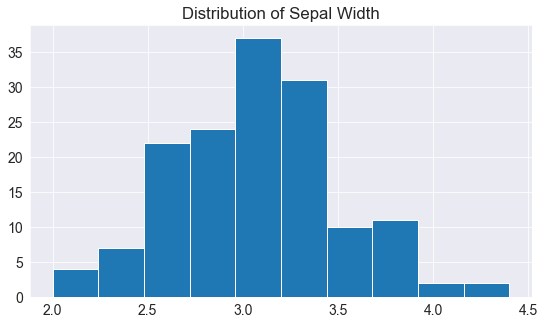We can immediately see that values of sepal width fall in the range 2.0 - 4.5, and around 35 values are in the range 2.9 - 3.1, which seems to be the largest bin.

#### Controlling the size and number of bins

We can control the number of bins, or the size of each bin using the `bins` argument.

In :
``````# Specifying the number of bins
plt.hist(flowers_df.sepal_width, bins=5);``````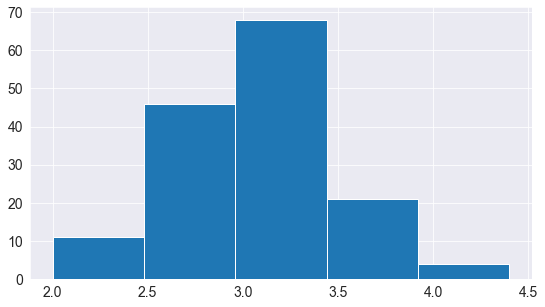In :
``````import numpy as np

# Specifying the boundaries of each bin
plt.hist(flowers_df.sepal_width, bins=np.arange(2, 5, 0.25));``````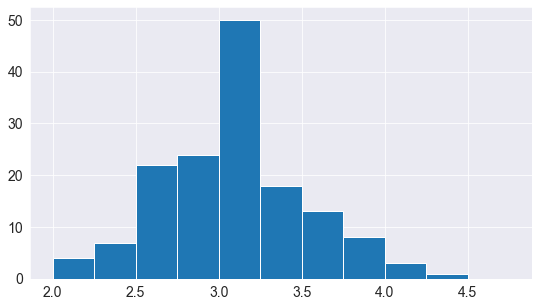In :
``````# Bins of unequal sizes
plt.hist(flowers_df.sepal_width, bins=[1, 3, 4, 4.5]);``````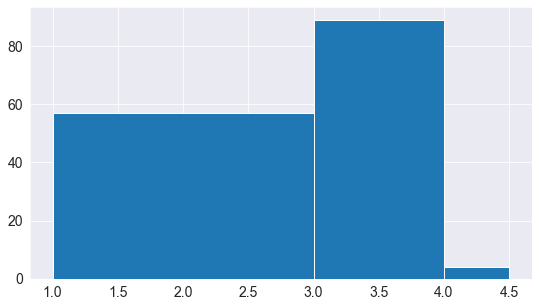#### Multiple Histograms

Similar to line charts, we can draw multiple histograms in a single chart. We can reduce the opacity of each histogram, so the the bars of one histogram don't hide the bars for others.

Let's draw separate histograms for each species of flowers.

In :
``````setosa_df = flowers_df[flowers_df.species == 'setosa']
versicolor_df = flowers_df[flowers_df.species == 'versicolor']
virginica_df = flowers_df[flowers_df.species == 'virginica']``````
In :
``````plt.hist(setosa_df.sepal_width, alpha=0.4, bins=np.arange(2, 5, 0.25));
plt.hist(versicolor_df.sepal_width, alpha=0.4, bins=np.arange(2, 5, 0.25));``````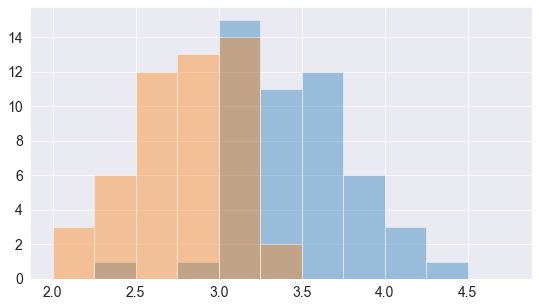We can also stack multiple histograms on top of one another.

In :
``````plt.title('Distribution of Sepal Width')

plt.hist([setosa_df.sepal_width, versicolor_df.sepal_width, virginica_df.sepal_width],
bins=np.arange(2, 5, 0.25),
stacked=True);

plt.legend(['Setosa', 'Versicolor', 'Virginica']);``````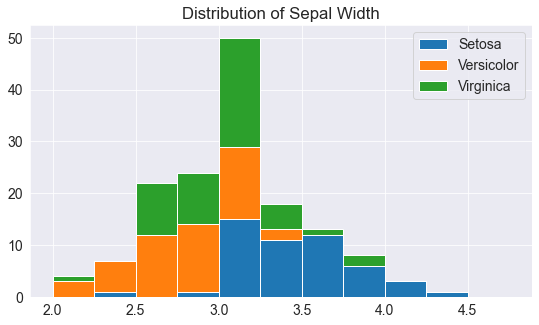Let's save and commit our work before continuing

In :
``import jovian``
In :
``jovian.commit()``
```[jovian] Attempting to save notebook.. [jovian] Updating notebook "aakashns/python-matplotlib-data-visualization" on https://jovian.ml/ [jovian] Uploading notebook.. [jovian] Capturing environment.. [jovian] Committed successfully! https://jovian.ml/aakashns/python-matplotlib-data-visualization ```
Out:
``'https://jovian.ml/aakashns/python-matplotlib-data-visualization'``

### Bar Chart

Bar charts are quite similar to line charts i.e. they show a sequence of values, however a bar is shown for each value, rather than points connected by lines. We can use the `plt.bar` function to draw a bar chart.

In :
``````years = range(2000, 2006)
apples = [0.35, 0.6, 0.9, 0.8, 0.65, 0.8]
oranges = [0.4, 0.8, 0.9, 0.7, 0.6, 0.8]``````
In :
``plt.bar(years, oranges);``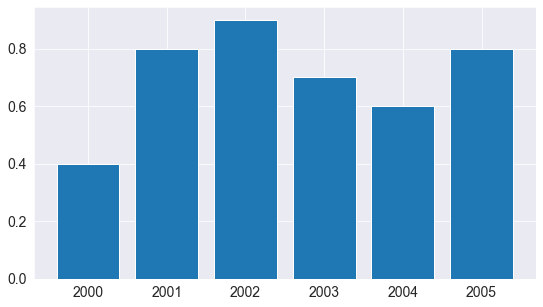Like histograms, bars can also be stacked on top of one another. We use the `bottom` argument to `plt.bar` to achieve this.

In :
``````plt.bar(years, apples)
plt.bar(years, oranges, bottom=apples);
``````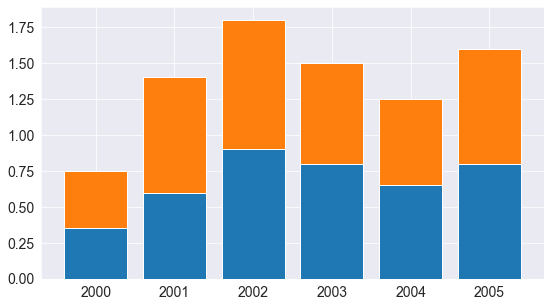#### Bar Plots with Averages

Let's look at another sample dataset included with Seaborn, called "tips". The dataset contains information about the sex, time of day, total bill and tip amount for customers visiting a restaurant over a week.

In :
``tips_df = sns.load_dataset("tips");``
In :
``tips_df``
Out:

We might want to draw a bar chart to visualize how the average bill amount varies across different days of the week. One way to do this would be to compute the day-wise averages and then use `plt.bar` (try it as an exercise).

However, since this is a very common use case, the Seaborn library provides a `barplot` function which can automatically compute averages.

In :
``sns.barplot('day', 'total_bill', data=tips_df);``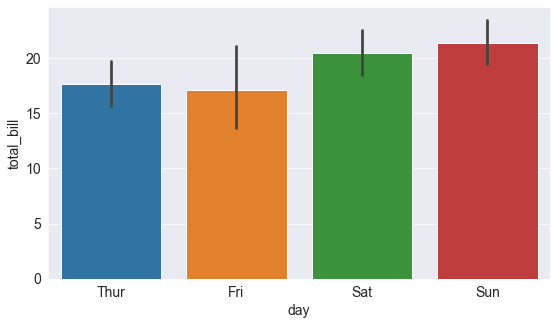The lines cutting each bar represent the amount of variation in the values. For instance, it seems like the variation in the total bill was quite high on Fridays, and lower on Saturday.

We can also specify a `hue` argument to compare bar plots side-by-side based on a third feature e.g. sex.

In :
``sns.barplot('day', 'total_bill', hue='sex', data=tips_df);``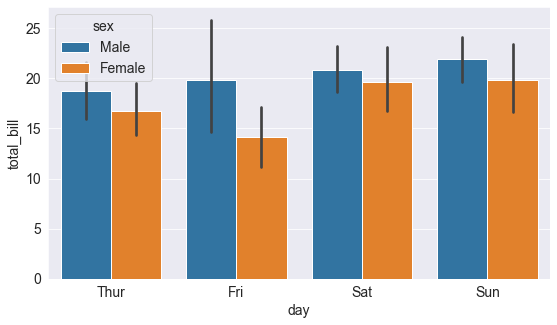You can make the bars horizontal simply by switching the axes.

In :
``sns.barplot('total_bill', 'day', hue='sex', data=tips_df);``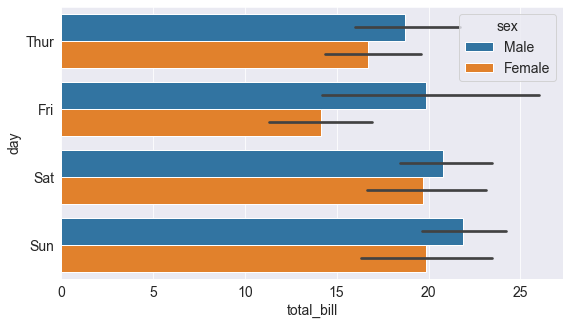Let's save and commit our work before continuing

In :
``import jovian``
In :
``jovian.commit()``
```[jovian] Attempting to save notebook.. [jovian] Updating notebook "aakashns/python-matplotlib-data-visualization" on https://jovian.ml/ [jovian] Uploading notebook.. [jovian] Capturing environment.. [jovian] Committed successfully! https://jovian.ml/aakashns/python-matplotlib-data-visualization ```
Out:
``'https://jovian.ml/aakashns/python-matplotlib-data-visualization'``

### Heatmap

A heatmap is used to visualize 2-dimensional data like a matrix or a table using colors. The best way to understand it is by looking at an example. We'll use another sample dataset from Seaborn, called "flights", to visualize monthly passenger footfall at an airport over 12 years.

In :
``flights_df = sns.load_dataset("flights").pivot("month", "year", "passengers")``
In :
``flights_df``
Out:

`flights_df` is a matrix with one row for each month and one column of each year. The values in the matrix show the number of passengers (in thousands) that visited the airport in a specific month of a specific year. We can use the `sns.heatmap` function to visualize the footfall at the airport.

In :
``````plt.title("No. of Passengers (1000s)")
sns.heatmap(flights_df);
``````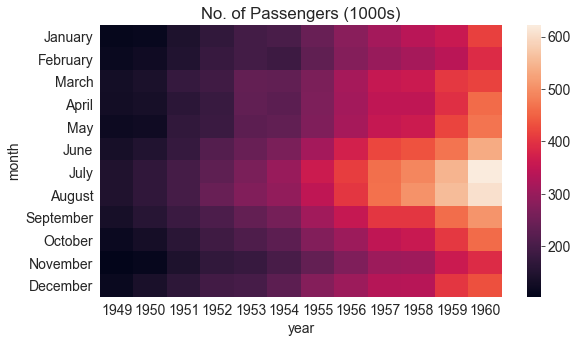The brighter colors indicate a higher footfall at the airport. By looking at the graph, we can infer two things:

• The footfall at the airport in any given year tends to be the highest around July & August.
• The footfall at the airport in any given month tends to grow year by year.

We can also display the actual values in each block by specifying `annot=True`, and use the `cmap` argument to change the color palette.

In :
``````plt.title("No. of Passengers (1000s)")
sns.heatmap(flights_df, fmt="d", annot=True, cmap='Blues');``````